Mechanics

# gravity acceleration

Gravity is the acceleration produced from a gravitational force of attraction. The gravity of a star, such as the Earth or the Sun , can be calculated based on the law of universal gravitation . The gravitational acceleration produced by a body is proportional to its mass and the constant of universal gravitation, and also inversely proportional to the square of the distance to the body’s center of mass. In addition, when a body falls towards the Earth, exclusively by the effect of gravity, we say that it is a free- fall movement .

## What is gravity?

The Sun’s gravity keeps the Earth in its orbit, similarly the Earth’s gravity keeps the Moon revolving around us. The Earth’s gravity is also responsible for maintaining atmospheric gases and for keeping all living beings attached to its surface. The intensity of this gravity, measured at sea level, is approximately 9.8 m/s², however, this value can vary according to height.

The Earth’s gravity is the same for all bodies that are at the same height on its surface , that is, free from the forces of air resistance and any other dissipative forces, all bodies that are dropped will fall towards the center of the Earth. and they will hit the ground at exactly the same time.

To understand why bodies of different masses fall towards the Earth at equal times, it is necessary to understand that the weight force that acts on each of them is different. Bodies of greater mass will present greater weights, but, at the same time, will have greater inertia , that is, they will present greater resistance to the variation of velocity.

## How to calculate the acceleration of gravity?

The calculation of gravitational acceleration is done through the law of universal gravitation , coined by the English physicist Isaac Newton . According to this law, the gravitational force that arises between bodies that have mass is always attractive and can be calculated based on the following mathematical expression: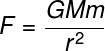F – gravitational force of attraction or weight force (N)

M in – masses of bodies (kg)

G – universal gravitation constant (6,674.10 -11 m 3 /kg.s²)

r – distance between bodies (m)

When a gravitational force such as the one shown in the equation acts on a body of mass m, an acceleration will be produced in the same direction as that force . According to Newton’s second law, the net force on a body is equal to its mass multiplied by its acceleration, check: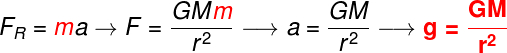According to the calculation shown, obtained based on the law of universal gravitation, it was possible to obtain an expression for the calculation of gravitational acceleration (g):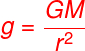With this last formula, we will use the mass of the Earth (M = 5.972.10 24 kg) and its average radius (r = 6371 km or 6.371.10 6 m) to estimate its gravity, check: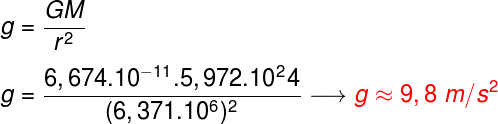Based on the formula used to calculate gravity on the Earth’s surface, we can estimate it for certain heights in relation to sea level.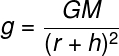If we make a calculation of the acceleration of gravity at heights of 400 km in relation to the Earth’s surface — quite common for orbiting vehicles such as space stations and satellites — we will find a gravity 10% less than the local gravity. You may be wondering how astronauts float when they are inside these ships, and the answer has to do with the concept of weightlessness.

When space vehicles are orbiting the Earth, they fall towards its center with the acceleration of gravity, however, their high tangential velocity makes their distance from Earth not change. Furthermore, gravitational acceleration plays the role of centripetal acceleration , which leads us to a curious situation. It turns out that, during the orbital movement, the gravitational acceleration (centripetal) and the satellite’s velocity (tangential) form an angle of 90º with each other.

According to the definition, when the force and the displacement are perpendicular (90º) there will be no work done, this implies that the satellite’s velocity module does not change, but its direction. If a satellite orbits the Earth at a height of 400 km, being subjected to a gravity of approximately 8 m/s², its orbital speed remains constant, however, the direction of this speed relative to Earth changes at a rate of 8 radians. per second

The following figure, taken from Isaac Newton’s main work, Principia , illustrates bodies orbiting the Earth . If we neglect the dissipative forces and launch these bodies in a horizontal direction with a sufficiently large velocity, they will describe an orbit around the Earth. However, if we exceed too much this speed, called orbital speed, the trajectory of these bodies would be more open and they could even leave Earth orbit.

## Gravity acceleration on other planets

The acceleration of gravity of planets depends on their radius and also on their mass, check the value of gravity on the surface of planets and other celestial bodies in the Solar System :

• Mercury: 3.7 m/s²
• Venus: 8.87 m/s²
• Land: 9.80 m/s²
• Moon: 1.6 m/s²
• Mars: 3.711 m/s²
• Jupiter: 24.79 m/s²
• Saturn : 10.44 m/s²
• Uranus: 8.87 m/s²
• Neptune: 11.15 m/s²
• Pluto: 0.62 m/s²

## origin of gravity

According to Albert Einstein ‘s theory of general relativity , gravity arises due to the curvature of space-time . The equations of relativity suggest that the geometry of spacetime is altered when in the presence of very massive objects such as planets , stars and black holes . Look at the figure, which shows the effect of the mass of bodies on the fabric of space-time:

## Solved exercises on acceleration of gravity

Question 1) Determine the magnitude of the acceleration of gravity of Mars, knowing that its average radius is 3400 km (3.4 x 10 6 m) and that its mass is 6.4 x 10 23 kg.

Data: G = 6.7.10 -11 Nm²/kg²

a) 5.20 m/s²

b) 3.71 m/s²

c) 9.8 m/s²

d) 4.15 m/s²

e) 12.7 m/s²

Template: letter B

Resolution:

To calculate the gravity of a planet, we need its mass and radius, having these data in hand, we make the following calculation: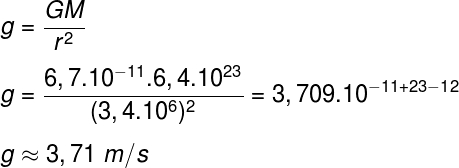According to the previous calculation, the acceleration due to gravity near the surface of Mars is 3.71 m/s².

Question 2) Determine how far a satellite needs to be from the Earth’s surface, where g = 10 m/s², so that the gravity acting on it is equal to 5 m/s².

Data:

Earth’s Radius: 6.4 x 10³ km

Earth Mass: 5.9 x 10 24 kg

a) 9020 km

b) 2920 km

c) 13600 km

d) 1500 km

e) 600 km

Template : Letter B

Resolution :

Using the formula to calculate gravity, we will find out what distance, in relation to the Earth’s surface, the satellite needs to be so that the gravity acting on it is equal to 5 m/s², check: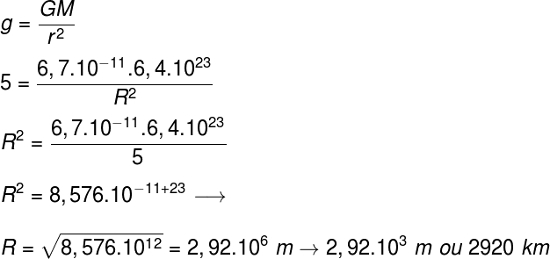By calculations, the Earth’s gravity will have a magnitude of 5.0 m/s² when we are at a distance of 2920 km from its surface.